# Synthetic Division - A Shortcut for Long Division!

##By PatrickJMT

This video demonstrates how to perform synthetic division of polynomials.# Fallacies: Fallacy of division

##By WiPhi

In this video, Paul Henne describes the fallacy of division, the informal fallacy that arises when we assume that the parts of some whole must have the same properties as the whole they make up. He also discusses why water molecules aren't wet. Speaker: Paul Henne# [4.NBT.6-1.0] Four digit division - Common Core Standard

##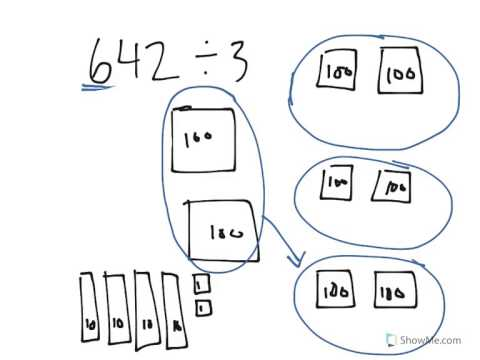By Freckle education

Find whole-number quotients and remainders with up to four-digit dividends and one-digit divisors, using strategies based on place value, the properties of operations, and/or the relationship between multiplication and division# Running distance in a week | Multiplication and division

##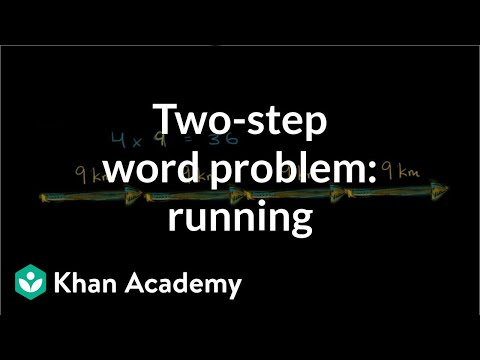By Khan Academy

Solve a two-step word problem by drawing a picture and creating an equation.# Total seats in a theater | Multiplication and division

##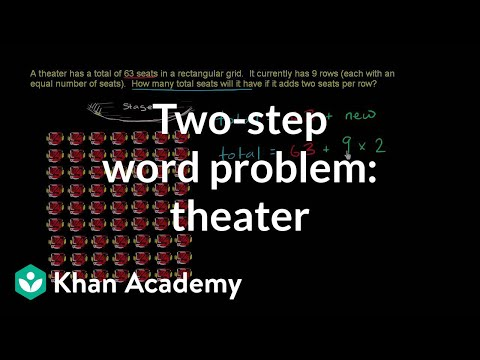By Khan Academy

Solve a two-step word problem by drawing a picture and creating an equation.# Marbles for friends | Multiplication and division

##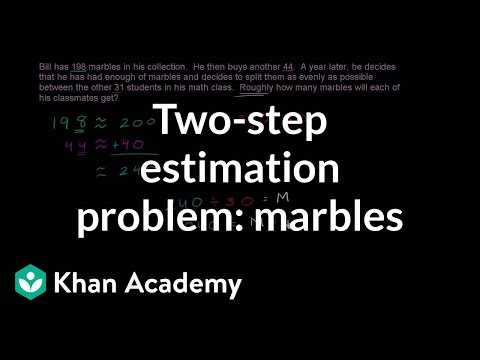By Khan Academy

Solve a two-step estimation word problem.# 4.NBT.6 - Division with Remainder (1-Digit Divisor)

##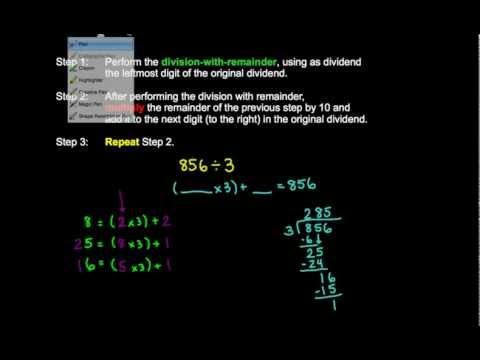By MathwithMrAlmeida

explains how to divide up to a 4-digit dividend by a 1-digit divisor to illustrate# Lesson 6.2 (Part 2) - Synthetic Division With A Binomial (A Not 1)

##By MrRainsberg

In this video lesson, we examine how to divide a polynomial by a binomial that does not have a leading coefficient of 1. This video contains several example problems.# Fractions are Division

##By mathantics

A fraction is just a division problem written like a number. This video also reviews the parts of a fraction and introduces the idea of decimals.# One-step equations with multiplication and division

##By Khan Academy

Remember that what you do to one side, you have to do to the other. Will you multiply or divide both sides to dump the fraction, x/a? Let's do it together.# One-step equations with multiplication and division

##By Khan Academy

This equation can be simplified through a single step to solve for the variable. Can you help?# One-step equations with multiplication and division

##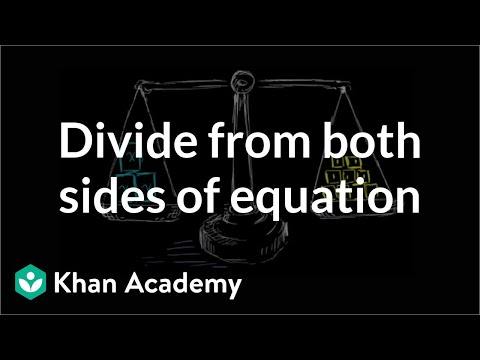By Khan Academy

Let's get a conceptual understanding of why one needs to divide both sides of an equation to solve for a variable.# One-step equations with multiplication and division

##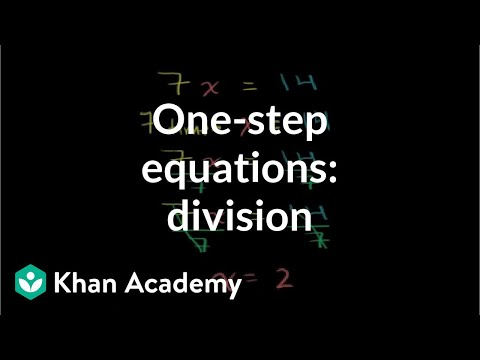By Khan Academy

Let's ease into this, shall we? Here's an introduction to basic algebraic equations of the form ax=b. Remember that you can check to see if you have the right answer by substituting it for the variable!# Introduction to Division

##By northstar15

Learn the basics of division including vocabulary and how to solve simple division problems. A teacher is using a whiteboard to explain the process as well as the vocabulary. He demonstrates two ways to write these division problems. Sound quality is poor.# Long Division

##By mathantics

Long Division# Multiplication and Division Fact Families

##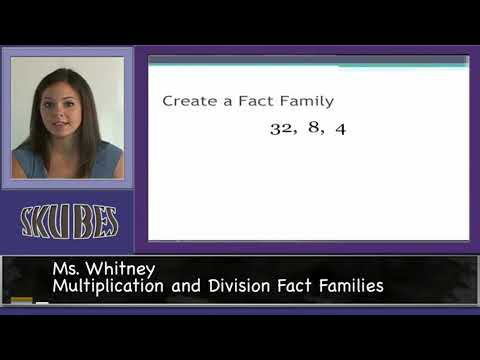By Skubes ed

3.OA.7 Fluently multiply and divide within 100, using strategies such as the relationship between multiplication and division (e.g., knowing that 8 × 5 = 40, one knows 40 ÷ 5 = 8) or properties# Multiplication and Division Relationships - Fun Math Videos for Kids 3rd Grade

##By Math&learning videos

Multiplication and division relationships# Unknowns with multiplication and division | Multiplication and division | 3rd grade | Khan Academy

##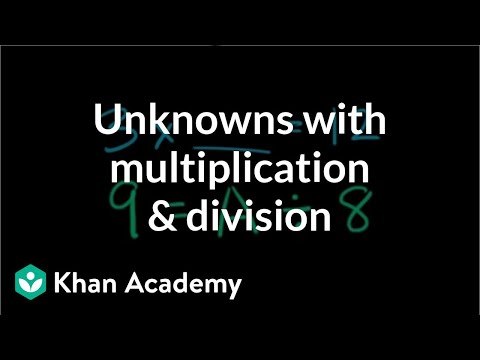By Khan Academy

Division of whole numbers# Dividing / Division of Integers

##By PatrickJMT

This video reviews the rule of signs. It then solves several multiplication and division problems using positive and negative integers.# Connection between multiplication and division - 3rd grade math lesson

##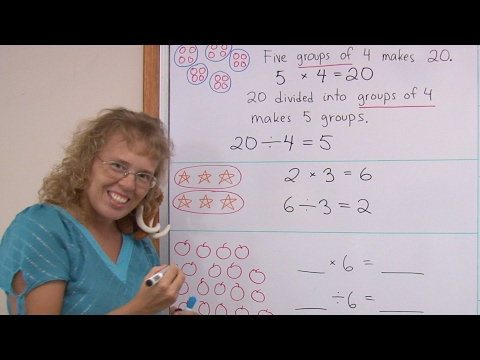By mathmamoth

For example, to solve 42 / 6, think how many times 6 makes 42 (in your six times table).